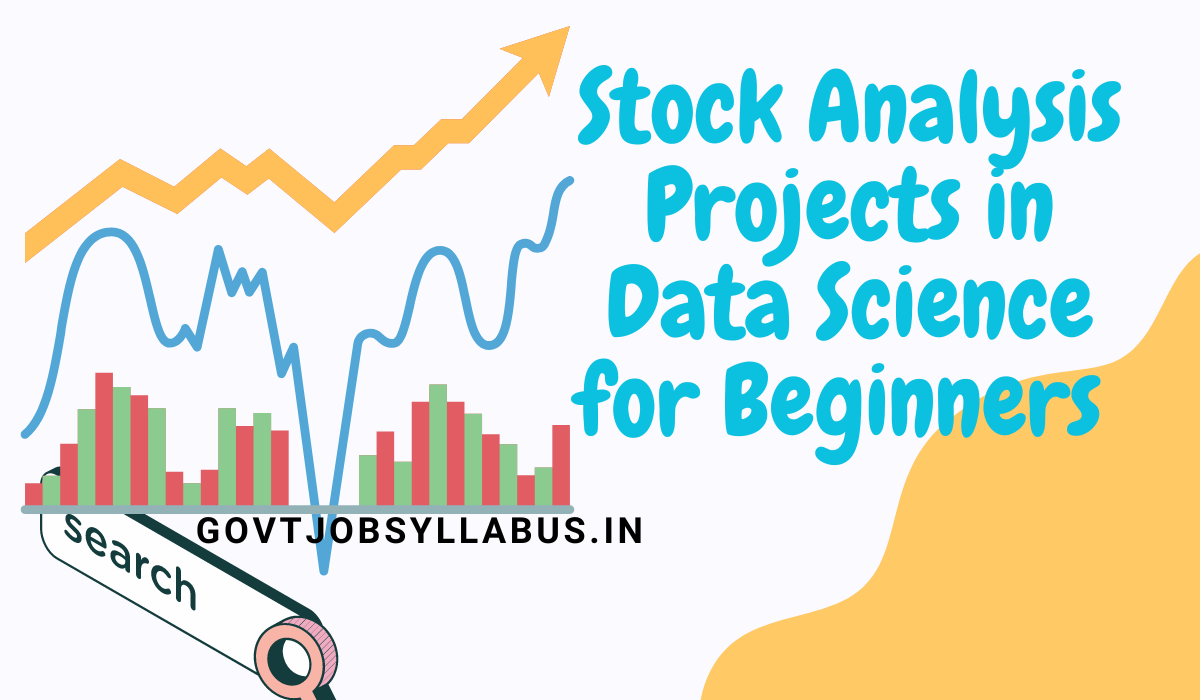Welcome again to our website govtjobsyllabus.in today we are going to add a data science project for beginners so if you are learning data science then you should check out our post because in this post we add a simple stock analysis project for data science.

You guys can try this project and learn the basic of data visualization.

## Data Science Projects For BeginnersStock Analysis Projects in Data Science for Beginners

Let's start to code:

import pandas as pd import numpy as np import matplotlib.pyplot as plt # Read the stock data from a CSV file df = pd.read_csv('stock_data.csv') # Convert the 'Date' column to datetime type df['Date'] = pd.to_datetime(df['Date']) # Set the 'Date' column as the index df.set_index('Date', inplace=True) # Calculate daily returns df['Daily Returns'] = df['Close'].pct_change() # Calculate moving averages df['50-day MA'] = df['Close'].rolling(window=50).mean() df['200-day MA'] = df['Close'].rolling(window=200).mean() # Plot the closing price and moving averages plt.figure(figsize=(10, 6)) plt.plot(df.index, df['Close'], label='Close') plt.plot(df.index, df['50-day MA'], label='50-day MA') plt.plot(df.index, df['200-day MA'], label='200-day MA') plt.xlabel('Date') plt.ylabel('Price') plt.title('Stock Analysis') plt.legend() plt.show()

• Let me tell you that if you guys don't have any historical data then you should use this code.

import yfinance as yf import pandas as pd import matplotlib.pyplot as plt # Fetch stock data using the ticker symbol and date range ticker = 'AAPL' start_date = '2022-01-01' end_date = '2022-12-31' data = yf.download(ticker, start=start_date, end=end_date)

ticker:- Type the stock name (We add the apple stock name in this example.)

### Explanation of data science projects for beginners

1.) Import the required libraries like I did in our program.

2.) Open CSV file or Use yfinance to extract data.

3.) Then set the data column as index.

df.set_index('Date', inplace=True)

4.) df['Daily Returns'] = df['Close'].pct_change()

In this code we use pct_change() that function will help to take the percentage change between consecutive closing prices.

5.)

df['50-day MA'] = df['Close'].rolling(window=50).mean()

df['200-day MA'] = df['Close'].rolling(window=200).mean()

This code will take the moving average of 50-day and 200-day using the rolling() function and then that value will be stored in two new columns '50-day MA' and '200-day MA'.

6.) Now plot the graph between the closing price and moving averages using this code :

plt.figure(figsize=(10, 6)) plt.plot(df.index, df['Close'], label='Close') plt.plot(df.index, df['50-day MA'], label='50-day MA') plt.plot(df.index, df['200-day MA'], label='200-day MA') plt.xlabel('Date') plt.ylabel('Price') plt.title('Stock Analysis') plt.legend() plt.show()

#### Conclusion

Here in this post, we explain everything about stock analysis projects in data science so if you are searching for a data science project in Python to try out your skill then you should read our post till the end.